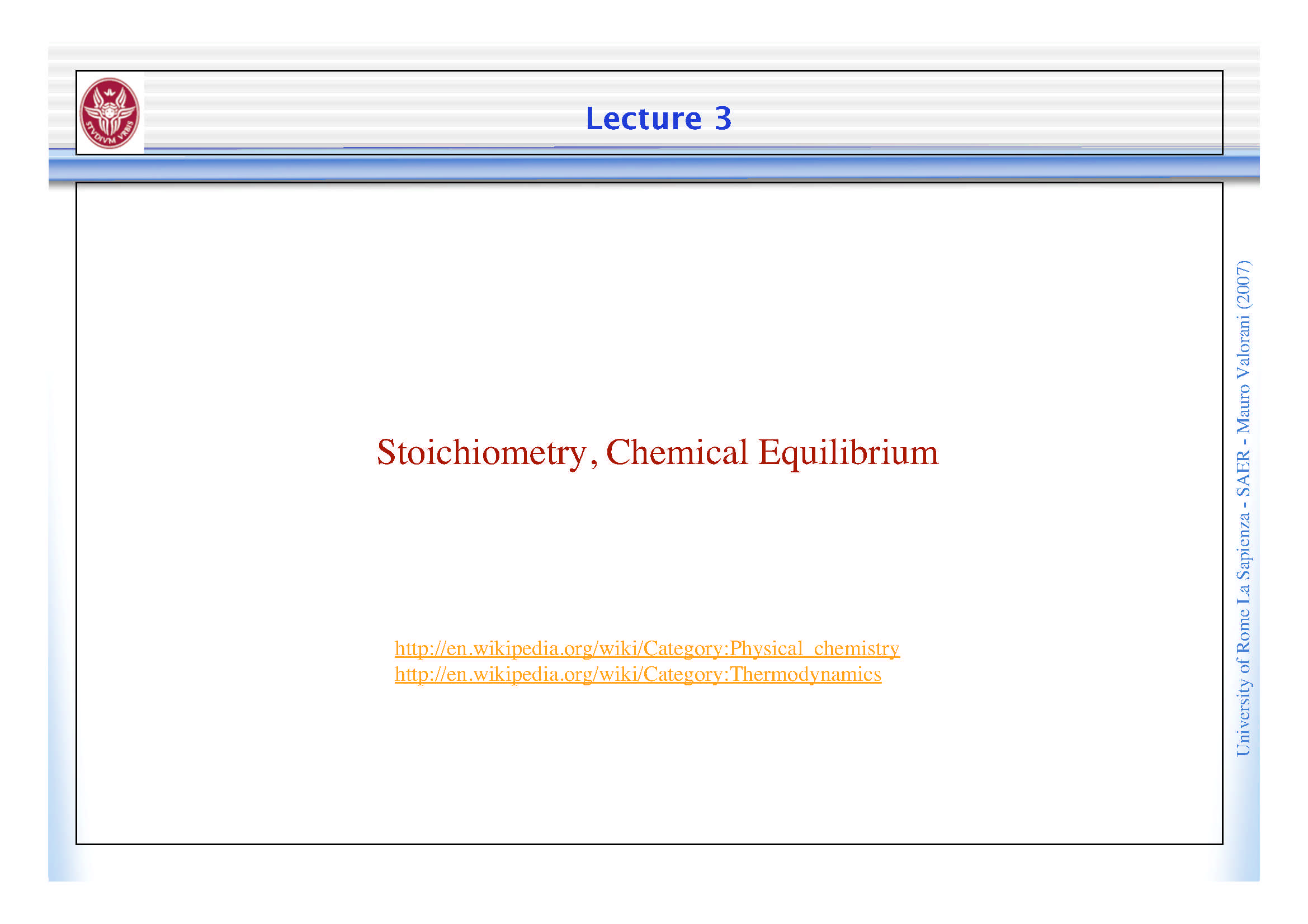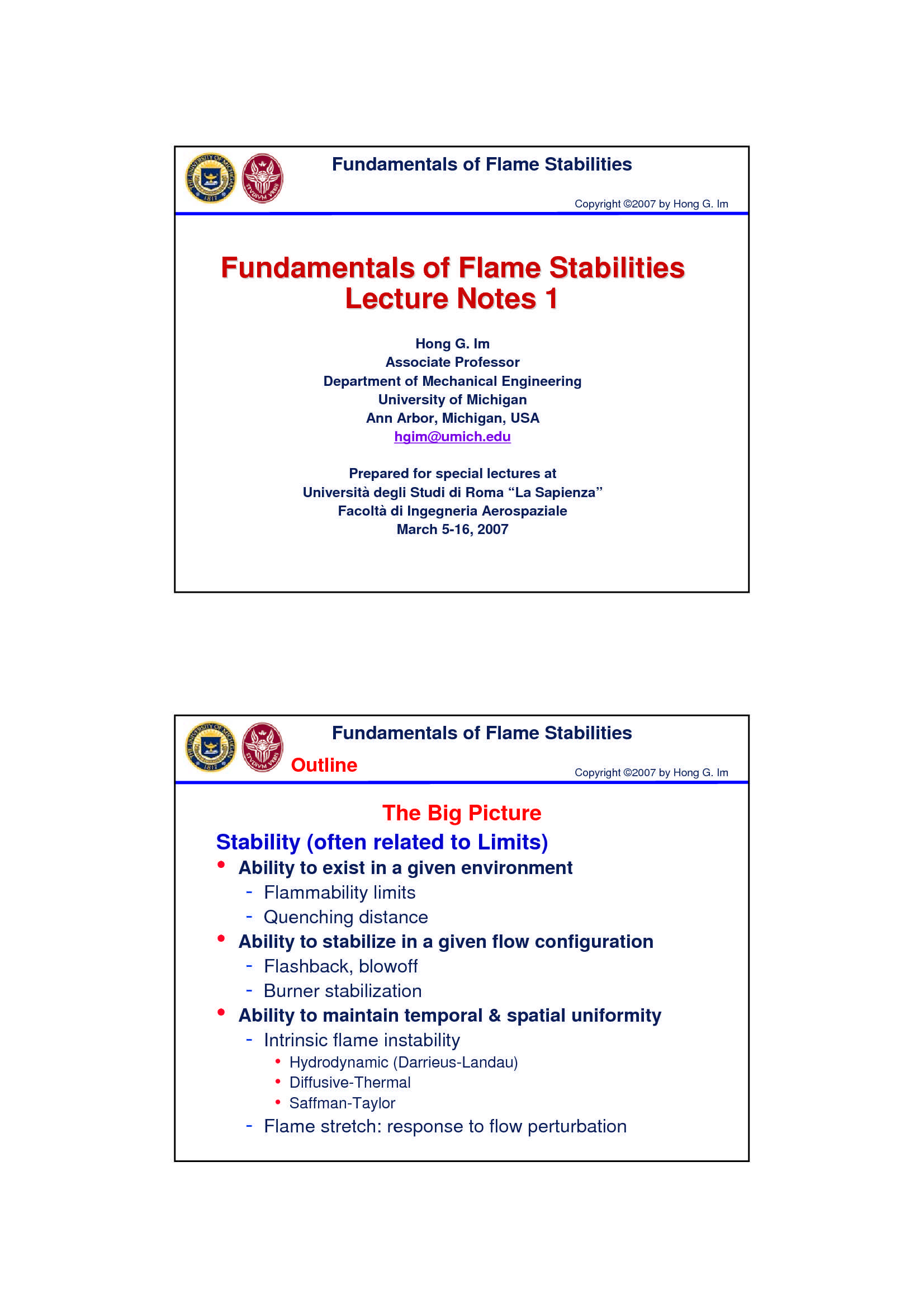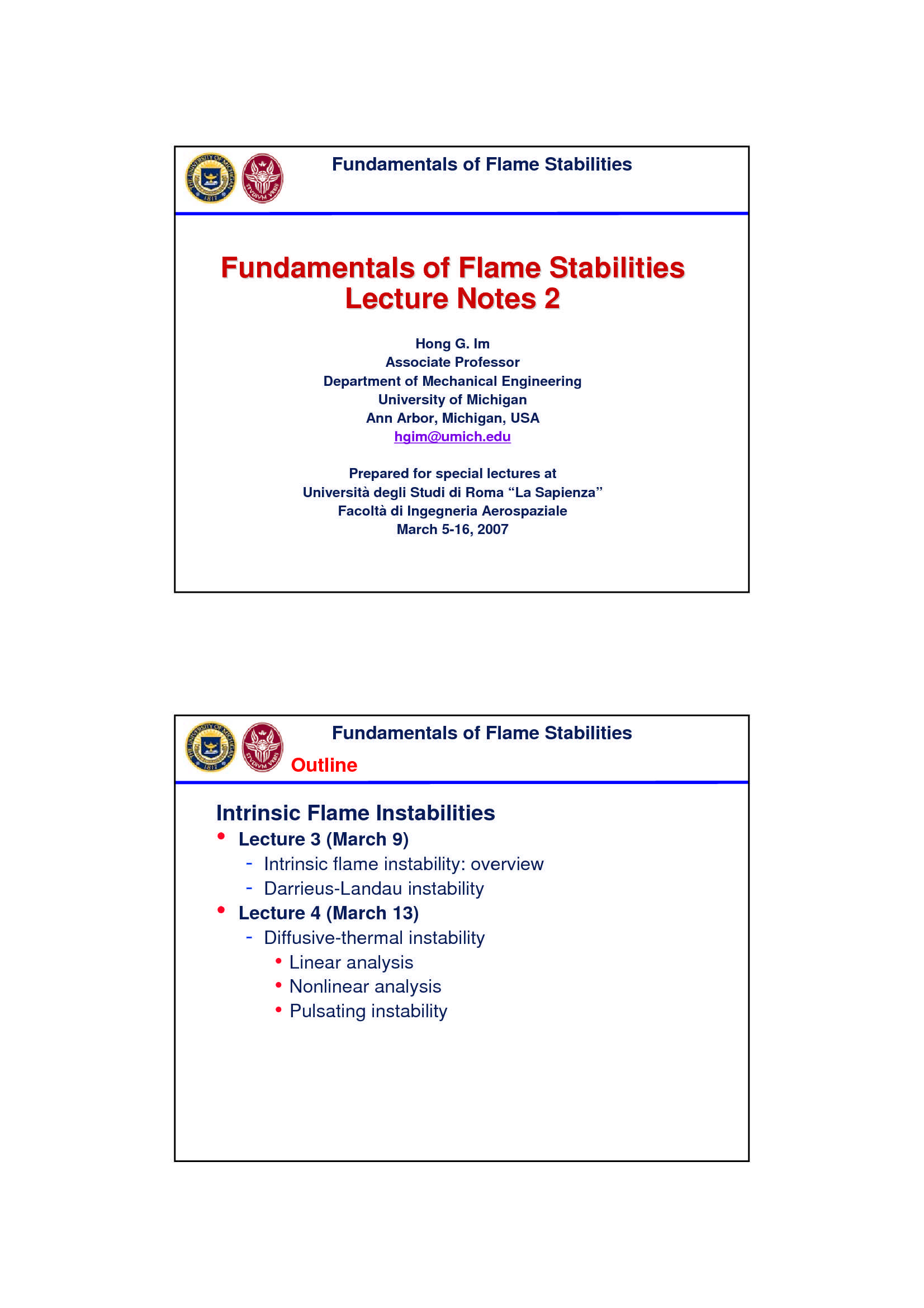Che materia stai cercando?

# Analisi di sensitività

Materiale didattico per il corso di Combustione del Prof. Mauro Valorani, all'interno del quale sono affrontati i seguenti argomenti: riduzione di modelli, analisi di sensitività; densità; il calcolo della sensitività; equilibrio parziale; modelli ridotti, il caso lineare; problemio della densità.

Esame di Combustione docente Prof. M. Valorani

Anteprima

### ESTRATTO DOCUMENTO

Model Reduction via QSSA & PEA

Having identified:

• (2007)

the species in (the production and destruction rates are

w in approximate balance) Valorani

partial equilibrium

the reaction in (the forward and the reverse reaction

w rates are in approximate balance) Mauro

-

## SAER

Makes possible the:

removal of species in , which generates a reduced

w -

Sapienza

model with a dimension lower than the original system

partial equilibrium

removal of reaction in , which might generate a reduced

w La

model with a lower stiffness and/or degree of nonlinearity than the Rome

original system of

University

Problem: how can we identify which reactions are in PEA or species in QSS?

Linear Systems & Exact Solution (2007)

! Valorani

dx =

# Ax

" dt Mauro

# =

x(0) x

$- 0 SAER = At Exact Solution: x(t) e x - 0 Sapienza ( ) dx d = = = At At Proof: e x Ae x Ax La 0 0 dt dt Rome of University Eigen-Systems & Matrix Exponent " # & 0 0 1 (2007) % ( " ) ! ! = ! = ! ii i Eigenvalues matrix of A : 0 0 ; i,j=1,N % ( j " Valorani$ '

0 0 N "

=

i ii i

Left eigenvector matrix L : LA=!L ; l A l i=1,N Mauro

"

= jj

Right eigenvector matrix R: AR=R! ; Ar r i=1,N -

j j SAER

* "

= j j

Decomposition of A: A=R!L r l

j j -

Sapienza

=1,

j N !

, ,

h h

* *

!

+ +

## A

Matrix Exponent: e ; e La

h! h! Rome

= =

h 0 h 0

# &

"

e 0 0 of

1 University

% (

! !

= = !

## A

e Re L e 0 0

% (

% (

"

$' 0 0 e N Invariant Directions & Characteristic Time Scales ! dx = (2007) # Ax " dt Valorani # = x(0) x$ 0 = Mauro

At

Exact Solution: x(t) e x 0 -

## SAER

=

Let x r , with r an eigenvector of A: -

0 k k Sapienza

( )

& &

' ' '

(

%t

= = = = = =

t t

ir

At At j ki t

x(t) e x e r Re Lr r e l r e r e

j j k

0 k k j k j k La

=1, =1,

j N j N Rome

Thus, for all times, x(t) has the same direction of x 0 of

' t University

e is the change in magnitude of the solution along r

k k

1

' ' ) )

= = * =

t

e 1 for 1 is a characteristic time scale along r

k '

k k k k

k

Stable, Unstable and Central Time Scales & Subspaces

=

Let x r , with r an eigenvector of A:

0 k k (2007)

!

== t

x(t) r e k

k

! = " = Valorani

if 0 x(t) r x(t) is invariant: x(t) =const, r =const

k k k

!

! ! #

= # < " = t

if 0 x(t) r e x(t) / x(0) <1 contraction

k k Mauro

!

! ! +

= + > " = t

if 0 x(t) r e x(t) / x(0) >1 expansion

k k -

## SAER

! = "

if 0 r is an invariant (central) direction -

Sapienza

k k

! < "

if 0 r is a stable direction

k k

! > "

if 0 r is an unstable direction La

k k Rome

of

= 

## C S U C

=

E {Subspace spanned by stable eigenvectors}

S =

E {Subspace spanned by unstable eigenvectors}

U =

E {Null Space of A}

C Fast and Slow Time Scales & Subspaces

! " #

Let = +i be a complex eigenvalue:

i i i (2007)

Valorani

! ! ! !

> > > >

… +1

!

##

"

##

$! # " #$

1 M M N Mauro

fast scales slow scales -

= 

## E E E E -

stable fast slow C Sapienza

=

E {Subspace spanned by fast eigenvectors}

fast La

=

E {Subspace spanned by slow eigenvectors} Rome

slow of

University

Fast subspace is spanned by M basis vectors : r ,…, r

1 M

Slow subspace is spanned by N-M basis vectors : r ,…, r

M+1 N

Fast & Slow Time Scales: an example

Consider a 2-d ODE linear problem:

• ! $!$ ! $!$ y 2

y y y

d ( (2007)

!

= = =

0 i

## &

# & # & # &

A (0) A r (' )l

& i i

" % " % " %

dt z

z z z " % i=1

0 Valorani

With exact solution

• ) , ) , ) , Mauro

! $!$ ! $!$

y y y y

2

/ ( ( (

' ' '

= = +

0 0 0

t i t 1 t 2

+ . + . + .

(t) r e l r e l r e l

# & # & # & # &

i 1 2

" % " % " % " %

i 1 2

z z z z

* - * - * - -

=1 SAER

i 0 0 0

If |λ >> |λ |:

• 1| 2 -

Sapienza

λ

for times < O(1/λ ), the solution evolves on a fast scale O(1/ )

w 1 1

λ

after a time ~ O(1/λ ), it evolves on a slow scale O(1/ )

w 1 2 La

Rome

r

For t=∞ , f(x*)=0, with x* the fixed point

• 2 of

University

! $!$ ! $!$

*

y y 0

y

d ' ( ' ( '

0 A 0

# & # & # & r

# &

" % " % " %

" %

* 1

z z 0

dt z

Fast/Slow Coordinates

= =

Let a R and b L (2007)

! ! ! !

= = " = =

ix ix

1 1 2 2

Introduce Lx so that x R l l Valorani

!

d dx #!

= = = #Lx =

L LAx r

dt dt Mauro

2

!

!

# &

i i i

dt =1 =1 =1

i i i Amplitude

Mode Mauro

( iAx

i i

f b -

## M N

dx ! !

= = +

r s

Ax a f a f -

Sapienza

r s

dt "#



% "

$#$

%

=1 = +1

r s M

fast slow La

Rome

## N

! =

i

Outer product between vectors (Order one tensors) generates order two tensors: a b I of

i University

=1

i )

=

ia

i i

Inner product between vectors (Order one tensors) generates order zero tensors (scalars): b j j

Amplitude Dynamics

!

( ) ( )

= n

Vector field : g x a f x

n (2007)

=1,

n N

( ) ( )

" ig

n n

Mode Amplitude : f x b x Valorani

( ) # k i

dg x g dx

! ( ) ( )

= =

Time derivative : J x g x Mauro

# i

dt x dt

=1,

i N -

## SAER

( )

ig

n

d b

n n

df db dg

= +

ig i

n

= b -

Sapienza

dt dt dt dt n

df "

! $n n ! db db n m = f n=1, N = + = + ig ig n n b Jg b J # & m dt La " % =1, dt dt m N Rome$ ' $' n db da ( + !$

n ! # + = * +

db ' n n n m

& )

b J a b Ja

& )

= + i

n m % (

b J a f

# & of

* - % (

m m m

dt dt

) ,

m

" %

dt University

=1,

m N

( +

n

db

'

= + n m

b J a f n=1, N

* -

) , m

dt

=1,

m N Ideal Basis for Linear Problems

n

df " ! n m (2007)

= f n=1, N

m

dt =1,

m N Valorani

$n d$ % $% & ' # = # = n n n mn J=A b a = 0 A = m m m m Mauro dt ( +$ n

d -

$% & ' ! = + = SAER n n mn * - ## A m m m ) , dt - Sapienza n df La " & ' ' mn m n Full Decoupling: = f = f n=1, N Rome m n dt =1, m N of ' <0 ' = . .. . # = if n n t n University Exact Solution : f (t) f (t )e lim f (t) 0 n n 0 #/ t A mode amplitude vanishes if the corresponding eigenvalue is negative Reduced Model: Linear Case 1 f (t )$ "

" #

! = !

1 1 0

At time t : f (t) f (t )e 1 1

1 0 (2007)

e

"

>

At time t :

1 Valorani

% dx #$= + ! ' 1 2 2 t a f (x(t)) a f (x(t)) a f (x(t ))e 2 1 2 2 0 & Slow Dynamics: dt Mauro ' " = x(0) x( ) ( 1 - = ! iAx 1 1 SAER Equation of state: f (x) b 0 - Sapienza Initial Condition of Slow Dynamics should satisfy the EoS! La { } Rome " " " = ! ) *SIM = = iAx( 1 1 1 f (x( )) b ) 0 x( ) x : f (x) 0 1 1 1 of University = SIM Slow Invariant Manifold SIMs for Non Linear Models (2007) Valorani Mauro - ## SAER - Sapienza La Rome of University Short-term Dynamics of a Singularly Perturbed Problem The canonic form of a SPP during the short-term dynamics is: (2007) " dy ! ! = f (y,z; )$ & ' n f ~ O(1)

y Valorani

dt !

# with : << 1 & '

dz m g ~ O(1)

$z ! = g(y,z; ) Mauro % dt - ## SAER where t is the fast time scale. - Sapienza < The reduced model valid during the short-term dynamics, for is an m- t O(ε), dimensional set of ODEs with y as parameter: La " dy Rome " ! ! y y 0$ $dt of & # # dz University = dz g(y ,z;0)$

$= % g(y,z;0) dt % dt Long-term Dynamics of a Singularly Perturbed Problem The canonic form of a SPP during the long-term dynamics is: (2007) # dy Valorani " = f (y,z; ) % ' ( n f ~ O(1) y ! d ! " " =$ t , << 1, , Mauro

'(

dz m g ~ O(1)

% z

" "

= g(y,z; )

& ! -

d SAER

where t is the slow time scale. -

Sapienza

>>

The reduced model for is a set of DAE s:

t O(ε) La

# Rome

dy

% " n - dimensional set of ODEs

f (y,z;0)

\$ ! of

d University

% m - dimensional set of AE

=

& 0 g(y,z;0)

The fast/slow SPP decomposition in the 0 limit

e !

The implicit constraint on the fast variables z can be explicited as: (2007)

= ! =

0 g(y,z;0) z h (y) h is a smooth function

0 0 Valorani

The graph of h defines a critical manifold M :

0 0

+m

= ! " = !

n

M {(y,z) : z h (y) , y K} Mauro

0 0

To each point p=(y, h (y)) in M is associated a fast fiber F 0P -

0 0 SAER

+m

= ! " ! " !

oP n m

F {(y,z) : z , p M } -

Sapienza

0

dy " f (y,z;0)

Points in M are fixed points for:

0 ! La

d Rome

D g(y,h (y);0)

If the real parts of the eigenvalues of the Jacobian matrix z 0 of

are all negatives, then M is asymptotically stable, and all solutions on F contract exponentially

0P University

0

toward p.

The fast/slow SPP decomposition for small and finite

e ε

Fenichel theory [MIM, Gol’dshtein, Sobolev,’88] guarantees that for small, there

,

exists a function h whose graph is a Slow Invariant Manifold (SIM) M (2007)

ε ε

+m

= " # = "

n

M {(y,z) : z h (y) , y K}

! ! Valorani

To each point p=(y, (y)) in M is associated a fast fiber F P

h ε ε ε

+m

= " # " # "

P n m Mauro

F {(y,z) : z , p M }

! !

dy #

" f (y,z; ) -

The slow dynamics on M is described by SAER

!

ε d -

fast contracting

The motion of any point on F decomposes into a component along the fiber,

P Sapienza

ε

slow component

F , and a governed by the motion of the point p belonging to the SIM, M

## P

ε ε. La

Rome

! !

= +

: 2

M is O(ε)-close to M h (y) h (y) + h (y) + h (y) ...

ε 0 ! 0 1 1 of

University

F is O(ε)-close to F

## P P

0

e Singularly Perturbed vs. Stiff Problems

It can be shown that the time scales of a Fast/Slow SPP system satisfies the

condition: (2007)

"

min( )

! = <<

fast 1 Valorani

"

max( )

slow Mauro

ε,

Therefore, for small a SPP is also Stiff -

## SAER

However, most of the interesting problems

are not cast in the Fast/Slow SPP form, with -

Sapienza

e

slow and fast variables and being

independent of time. La

Rome

The Computational Singular Perturbation

(CSP) method can handle Stiff/SP problems of

University

e

with slow and fast variables and being Stoichiometric H /O Mixture; 8

dependent of time! 2 2

species and temperature; 6 reactions.

PAGINE

40

PESO

3.25 MB

AUTORE

PUBBLICATO

+1 anno fa

DETTAGLI
Esame: Combustione
Corso di laurea: Corso di laurea magistrale in ingegneria aeronautica
SSD:
A.A.: 2007-2008

I contenuti di questa pagina costituiscono rielaborazioni personali del Publisher Atreyu di informazioni apprese con la frequenza delle lezioni di Combustione e studio autonomo di eventuali libri di riferimento in preparazione dell'esame finale o della tesi. Non devono intendersi come materiale ufficiale dell'università La Sapienza - Uniroma1 o del prof Valorani Mauro.

Acquista con carta o conto PayPal

Scarica il file tutte le volte che vuoi

Paga con un conto PayPal per usufruire della garanzia Soddisfatto o rimborsato

RecensioniTi è piaciuto questo appunto? Valutalo!

## Altri appunti di CombustioneDispensa

### Stechiometria ed equilibrio chimicoDispensa

### Stabilità della fiammaDispensa

### Combustione omogenea e combustione eterogeneaDispensa